Disclaimer: This is an example of a student written essay.

Any opinions, findings, conclusions or recommendations expressed in this material are those of the authors and do not necessarily reflect the views of UKEssays.com.

# Speech Recognition using Epochwise Back Propagation

 ✅ Paper Type: Free Essay ✅ Subject: Computer Science ✅ Wordcount: 3712 words ✅ Published: 12th Mar 2018

International Journal of Computer Applications (0975 – 8887) Volume *– No.*, ___________ 2013

Speech Recognition using the Epochwise Back Propagation through time Algorithm

Neelima Rajput S.K.Verma

Department of C.S.E. Department of C.S.E.

GBPEC, Pauri Gharwal, GBPEC, Pauri Gharwal,

Uttrakhand, India. Uttrakhand, India

ABSTRACT

In this paper, artificial neural networks are used to accomplish the English alphabet speech recognition. The challenging issue in the area of speech recognition is to design an accurate and effective speech recognition system. We implemented a new data classification method, where we use neural networks, which are trained using the calculated epoch values of audio signal. This method gave comparable result to the already implemented neural networks. In this paper, Back propagation neural network architecture used to recognize the time varying input data, and provides better accurate results for the English Alphabet speech recognition. The Epochwise Back Propagation through time (BPTT) algorithm uses the epoch values of input signal to train the network structures and yields the satisfactory results.

Keywords Artificial Neural Network, Back Propagation Neural Network, Epoch, Speech Recognition.

I INTRODUCTION

Speech recognition system enables the machine to understand the human speech and react accordingly. It allows the machine to automatically understand the human spoken utterances with the speech signal processing and pattern recognition. In this approach is the machine converts the voice signal into the suitable text or command through the process of identification and understanding. Speech recognition is emerges as a vast technology in current time. It also plays an important role in information theory, acoustics, phonetics, linguistics, and pattern recognition theory and neurobiology disciplines. speech recognition technology become a key technology in the computer information processing technology as there is rapid advancement in the software, hardware and information technology. The features of input audio signal are compared with the voice template stored in the computer database in speech recognition system by using the computer systems. Recognition results are mainly depends upon the matching techniques used for matching the audio signal characteristics. To improve the recognition rate and better recognition results neural networks are used.

If you need assistance with writing your essay, our professional essay writing service is here to help!

A neural network is a powerful tool which used to adapt and represent the complicated input outputs. Neural nets are basically interconnected networks of relatively simple processing units, or nodes that work simultaneously. They are designed to mimic the function of human neuron biological networks. The processing units of neural networks are termed as neurons. A neural network provides better results over the existing approaches in speech recognition systems .

2 BASICS OF NEURAL NETWORKS

The basics of neural networks are discussed as follows. There are many different types of neural networks, but they all have four basic and common attributes:

• Processing units

• Connections

• Computing procedure

• Training procedure

.

1. Processing Units

A neural network contains several processing units, which are roughly analogous to neurons in the human brain. All these units activate in parallel and perform the task simultaneously. Processing units are responsible for the overall computation; there is no any other unit for the corporation of their activity. Each processing unit computes a scalar function of all its local inputs at every moment of time and then further broadcast the result to their neighboring units . The units in a neural network are basically classified into input units, which used to receive data from the outside; hidden units, used to internally transform the data; and output units, which serve decisions or target signals.

2.2 Connections

All processing units in a neural network are organized in to a defined topology by a set of connections, or weights, shown as lines in a diagram. Each weight consist a real value, which ranging from – ∞ to + ∞. The value of a weight represents how much impact a unit has on its neighbor units a positive weight causes one unit to excite another, while a negative weight causes one unit to inhibit another. Weights of the processing units are usually one-directional (from input units towards output units), but it may be two-directional sometimes, especially when there is no distinction between input and output units.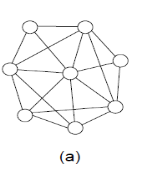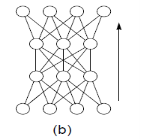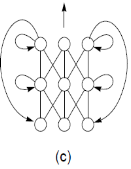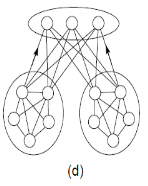Figure 1.Neural network topologies: (a) unstructured, (b) layered, (c) recurrent, (d) modular .

The above figure shows the topology of different neural networks. Unstructured Neural Network is mainly used in processor which is operated in parallel to provide the computational power for the speech recognition system. Layered neural net algorithms are self-organizing and their internal speech model maximizes the performance and produces better results comparable to existing systems.

Recurrent Neural network are mainly used in pattern sequencing as it follows the sequences changes time to time. Modular neural network are used in designing of complex systems by using simple components.

Neural nets offer an approach to computation that mimics biological nervous systems. Algorithms based on neural nets have been proposed to address speech recognition tasks which humans perform with little apparent effort.

2.3 Computation Procedure

Computation of neural networks begins after applying an input data to the input units of the network. Then the activation function of all the units is calculated either simultaneously or independently depends upon the structure of the neural network. The computation process in the unstructured network is termed as spreading activation and in layered network is known as forward propagation as it proceed from the input units to the output units. First we compute the net input of the processing unit and then we compute the output activation function of the net input to update a given processing unit.

2.4 Training Procedure

Training a network means enable the connections adaptive so that the network shows the actual computational behavior for all the input patterns. In training process usually weights are updated but sometimes the modification of network topology also takes place, i.e., addition and deletion of connections from the network topology . Modification of weight is easy and beneficial than topology modification as a network with bulk connections able to set any of its weight zero, which is equivalent as deleting such weights.

3. RELATED WORK

The past research concluded that the use of the neural networks in the speech recognition system provides the better recognition result compared to the other existing approaches. The latest study of neural networks actually started in the 19th century, when neurobiologists first introduce the extensive studies of the human nervous system . Cajal (1892) determined that the nervous system is comprised of some basic units i.e. discrete neurons, which communicate with the other neurons by sending electrical signals down their long axons, which ultimately activated and touch the dendrites(receptive areas) of thousands of other neurons, transmitting the electrical signals through synapses (points of contact, with variable resistance). Firstly, the different kinds of neurons were identified, and then analyze their electrical responses, and finally their patterns of connectivity and the brain’sgross functional areas were mapped out. According to the neurobiologists study the functionality of individual neurons are quite simple and easy. Whereas to determine how neurons worked together to achieve high level functionality, such as perception and cognition are very difficult.

In 1943 McCulloch and Pitts proposed the first enumeration model of a neuron, named as binary threshold unit, whose output was either 0 or 1 depending on whether its net input exceeded a given threshold. There are various approaches proposed by the researchers to design an accurate speech recognition system for various purposes. In  Al-Alaoui algorithm is used to train the neural network. This method gives the comparable better results to the already implemented hidden markov model (HMM) for the recognition of the words. This algorithm also overcomes the disadvantages of the HMM in the recognition of sentences. An algorithm based on neural network classifier  for speech recognition used a new Viterbi net architecture which is recognized the input patterns and provided an accuracy of recognition rate more than 99% on a large speech database. This system is used for isolated word recognizer. In  author accomplishes the isolated word speech recognition using the neural network. The methodology of this approach is to extract the feature of speech signals using the Digital Signal Processing techniques and then classification using the Artificial Neural Network. This algorithm concluded that the better accurate recognition results are obtained from the probalistic Neural Network PNN. In  author implemented a pre- trained deep neural network using the hidden markov model (DNN-HMM) hybrid architecture which is used to train the DNN to produce the better recognition results of large vocabulary speech database.

4. PROPOSED WORK

Speech recognition using the Epochwise Back propagation through time algorithm is proposed in this paper.In the proposed system neural network training is based on the calculation of epoch of the audio signal and then used these epoch value for the training of the neural network. The input data sets used to train the neural network can be partitioned in to the independent epochs. Each epoch representing a temporal value of the input data. Back propagationneural network used in the system in following steps.

1. First choose and fix the architecture for the network, which will contain input, hidden and output units, all of which will contain sigmoid functions.
2. Assign the weights between all the nodes. The assignments of weights usually between -0.5 and 0.5.
3. Each training example is used, one after another, to re-train the weights in the network.
4. After calculating each epoch for input audio data, a termination condition is checked.

In neural network architecture the weights of input and hidden layers are adjusted according to the target output values  The input data is considered as E which is propagated through the network so that we can record all the observed values Oi(E) for the output nodes Oi. At the same time, we record all the observed values hi(E) for the hidden nodes. Then, for each output unit Ok, we calculate its epoch as follows: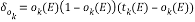(1)

The epoch terms from the output units are used to calculate epoch terms for the hidden units. In fact, this method gets its name because we propagate this information backwards through the network . For each hidden unit Hk, we calculate the epoch as follows: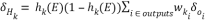(2)

Here, we take the epoch term for every output unit and multiply it by the weight from hidden unit Hk to the output unit. We then add all these together and multiply the sum by hk(E)*(1 – hk(E)). Having calculated all the epoch values associated with each unit (hidden and output), we can now transfer this information into the weight changes Δij between units i and j. The calculation is as follows: for weights wij between input unit Ii and hidden unit Hj, and summation of all units are as: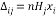(3)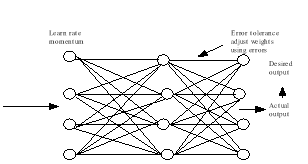Back Propagation Neural Network architecture is shown in below figure

Fig.2 Back propagation Neural Network

The main steps of this system are defined as follows.

1. Read the input audio Signal.
2. Extract the epoch values
3. Train the neural network on the basis of epoch values.
4. Applied the back propagation neural network for the classification.
5. Matching the input data with the trained data.
6. Recognized the input.

The data flow diagram of the proposed system represented in given figure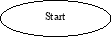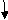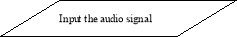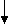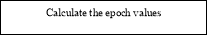C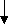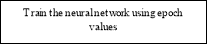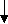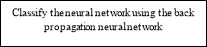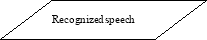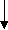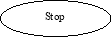Figure 2 Proposed approaches for Speech Recognition

5. EXPERIMENTAL RESULTS

The experiment conducted on the audio database of English alphabets. Matlab R2010a (Math works) in Windows 7 was used to implement the proposed algorithm. The input signal is used to calculate the epoch values and then by using calculated epoch values the neural network is trained.The epoch rate of multi-layered networks over a training set could be calculated as the number of miss-classified data values. There are many output nodes, all of which could potentially misfire (e.g., giving a value close to 1 when it should have output 0 and vice-versa), we can be more sophisticated in our epoch evaluation. In practice the overall network epoch is calculated as: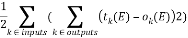This is not as complicated as it first appears. The calculation simply involves working out the difference between the observed output for each output unit and the target output and squaring this to make sure it is positive, then adding up all these squared differences for each output unit and for each input signal. The calculated epoch values for audio signal A is listed in tabular form.

Table: 1 Calculated Epoch values for input data

 Input data A Time in mili seconds Epoch value 1000 0.15518 2000 0.068235 3000 0.042088 4000 0.022866 5000 0.01843 6000 0.015408 7000 0.013233 8000 0.011595 9000 0.010317 10287 0.0099998

Then epoch values are basically instant of significant excitation of the vocal-tract system during production of speech. Table 1 represented the Epoch values for the input English alphabet A in terms of time in mili seconds. These epoch values are stored in the computer for the further matching by using the neural networks to the recognition purpose. Similarly, epoch values are calculated for each input English alphabet and then train the network through these input patterns and stored in the system for recognition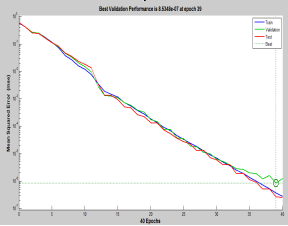Figure 3 Performance graphs of epoch values.

The figure 3 shows the performance of the system based on epoch values. The best validation performance epoch value is selected from the different epoch on the basis of Mean Square error. The graph is plotted on epoch and means squared error values.

Table: 2 Performance of the proposed system

 Input alphabate No of sound samples Recognition rate A 5 100% B 5 100 C 5 100% D 5 100% E 5 98% Total 99.6

In Table: 2 the recognition rate is calculated for five input English alphabet which is 98.8%. The new proposed Epochwise Back propagation through time algorithm yields the satisfactory results.

6. CONCLUSION

In this paper, we implemented the Epochwise Back propagation through the time varying epoch calculation. The experiment is conducted on the small set of English Language alphabet to calculate the recognition rate of the system. Some different sound samples (i.e., with different sampling frequency) of each alphabet are taken and used for testing the system. The above results show the performance of our proposed algorithm in speech recognition.

7. REFERENCES

1. Jianliang Meng, Junwei Zhang,Haoquan Zhao, “Overview of the speech Recognition Technology”, 2012 Fourth International Conference on Computational and Information Sciences.
1. L. Fausset, Fundamentals of Neural Networks. PrenticeHall Inc., 1994, ch 4.
1. Jiang Ming Hu, in the Yuan Baozong, Lin Biqin. Neural networks for speech recognition research and progress. Telecommunications Science, 1997, 13(7):1-6.
1. H. Boulard and N. Morgan, “Continuous speech recognition by connectionist statistical methods,” IEEE Trans. Neural Netw., vol. 4, no. 6, pp. 893–909, Nov. 1993.
1. Mohamad Adnan Al-Alaoui, Lina Al-Kanj, Jimmy Azar, and Elias Yaacoub, “Speech recognition using Artificial Neural Network and Hidden Markov Model”, IEEE Multidisciplinary Engineering Education Magazine Vol. 3, No.3, September 2008.
1. RICHARD P. LIPPMANN, “Neural Network Classifiers for Speech Recognition” The Lincoln Laboratory Journal, Volume 1, Number 1 (1988)
1. Gulin Dede, Murat Husnu SazlÄ±, “Speech recognition using artificial neural network.” Digital signal processing © 2009 Elsevier Inc.
1. George E. Dahl, Dong Yu, Li Deng, Alex Acero,” Context-Dependent Pre-Trained Deep Neural Networks for Large-Vocabulary Speech Recognition” , IEEE Transaction on Audio, and Language Processing, Vol. 20, No.1, January 2012.
1. H. Paugam-Moisy, ‘Parallel neural computing based on network duplicating’,in Parallel Algorithms for Digital Image Processing, Computer Vision and Neural Networks, ed., I. Pitas, 305–340, JohnWiley, (1993).
1. Stefano Scanzio, Sandro Cumani, Roberto Gemello, Franco Mana, P. Laface, “Parallel implementation of Artificial Neural Network Training for Speech Recognition.” Pattern recogonition letters, © 2010 Elsevier B.V.
1. N. Morgan and H. Bourlard, “Continuous speech recognition using multilayer perceptrons with hidden Markov models,” in Proc. ICASSP,1990, pp. 413–416.
1. Y. Hifny and S. Renals, “Speech recognition using augmented conditional random fields,” IEEE Trans. Audio, Speech, Lang. Process., vol.17, no. 2, pp. 354–365, Feb. 2009.

View all

## DMCA / Removal Request

If you are the original writer of this essay and no longer wish to have your work published on UKEssays.com then please:

Related Services

Prices from

KD47

Approximate costs for:

• 1000 words
• 7 day deliveryHumanity University

Dedicated to your worth and value as a human being!

Related Lectures